# Parseval equality

(diff) ← Older revision | Latest revision (diff) | Newer revision → (diff)

An equality expressing the square of the norm of an element in a vector space with a scalar product in terms of the square of the moduli of the Fourier coefficients of this element in some orthogonal system. Thus, ifis a normed separable vector space with a scalar product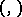, if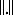is the corresponding norm and ifis an orthogonal system in,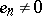,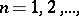then Parseval's equality for an elementis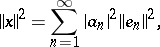(1)

where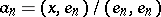,are the Fourier coefficients ofin the system. Ifis orthonormal, then Parseval's equality has the form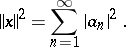The validity of Parseval's equality for a given elementis a necessary and sufficient condition for its Fourier series in the orthogonal system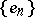to converge toin the norm of. The validity of Parseval's equality for every elementis a necessary and sufficient condition for the orthogonal systemto be complete in(cf. Complete system). This implies, in particular, that:

1) ifis a separable Hilbert space (cf. Hilbert space) andis an orthogonal basis of it, then Parseval's equality holds forfor every;

2) ifis a separable Hilbert space,, ifis an orthonormal basis ofand if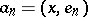and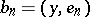are the Fourier coefficients ofand, then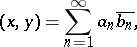(2)

the so-called generalized Parseval equality. In a fairly-definitive form the question of the completeness of a system of functions that are the eigen functions of differential operators was studied by V.A. Steklov in .

Parseval's equality can also be generalized to the case of non-separable Hilbert spaces: If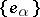,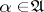(is a certain index set), is a complete orthonormal system in a Hilbert space, then for any elementParseval's equality holds: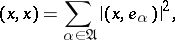and the sum on the right-hand side is to be understood aswhere the supremum is taken over all finite subsets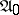of.

When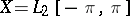, the space of real-valued functions with Lebesgue-integrable squares on, and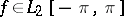, then one may take the trigonometric system as a complete orthogonal system and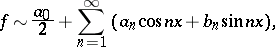where (1) takes the form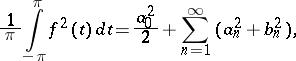which is called the classical Parseval equality. It was proved in 1805 by M. Parseval.

If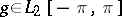andthen an equality similar to (2) looks as follows: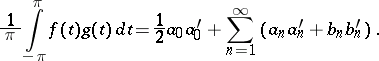(3)

Two classesand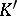of real-valued functions defined on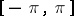and such that for all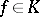and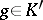Parseval's equality (3) holds are called complementary. An example of complementary classes are the spaces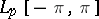and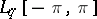,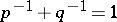,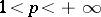.

How to Cite This Entry:
Parseval equality. Encyclopedia of Mathematics. URL: http://encyclopediaofmath.org/index.php?title=Parseval_equality&oldid=11840
This article was adapted from an original article by L.D. Kudryavtsev (originator), which appeared in Encyclopedia of Mathematics - ISBN 1402006098. See original article